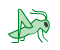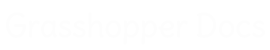﻿ Grasshopper Curve - Addon for Grasshopper | Grasshopper DocsCommunity documentation for Grasshopper add-ons & plugins# Grasshopper Curve

##### SYSTEM COMPONENTS. Version 1.0.0007.Released on 2022-Mar-08.Provides 106 components.Created by David Rutten.Features 0 video tutorials.
 Analysis
 Division
 Primitive
 Spline
 Util

### Analysis

 Evaluate Length (Eval)Evaluate a curve at a certain factor along its length. Length factors can be supplied both in curve units and normalized units. Change the [N] parameter to toggle between the two modes. Closed (Cls)Test if a curve is closed or periodic. Control Points (CP)Extract the nurbs control points and knots of a curve. Control Polygon (CPoly)Extract the nurbs control polygon of a curve. Curve Closest Point (Crv CP)Find the closest point on a curve. CurvatureEvaluate the curvature of a curve at a specified parameter. Derivatives (CDiv)Evaluate the derivatives of a curve at a specified parameter. Discontinuity (Disc)Find all discontinuities along a curve. Curve Domain (CrvDom)Measure and set the curve domain Extremes (X-tremez)Find the extremes (highest and lowest points) on a curve. Curve Middle (MidPt)Get the point in the middle of a curve Curve Nearest Object (CrvNear)Find the object nearest to a curve. Curve Proximity (CrvProx)Find the pair of closest points between two curves. Segment Lengths (LenSeg)Finds the shortest and longest segments of a curve. Curve Side (Side)Find on which side of a curve a point exists TorsionEvaluate the torsion of a curve at a specified parameter. Deconstruct Arc (DArc)Retrieve the base plane, radius and angle domain of an arc. Deconstuct Rectangle (DRec)Retrieve the base plane and side intervals of a rectangle. End Points (End)Extract the end points of a curve. Evaluate Curve (Eval)Evaluate a curve at the specified parameter. Length (Len)Measure the length of a curve. Length Domain (LenD)Measure the length of a curve subdomain. Length Parameter (LenP)Measure the length of a curve to and from a parameter. PlanarTest a curve for planarity. Point In Curve (InCurve)Test a point for closed curve containment. Point in Curves (InCurves)Test a point for multiple closed curve containment. Polygon Center (PCen)Find the center point (average) for a polyline. Curve Frame (Frame)Get the curvature frame of a curve at a specified parameter. Horizontal Frame (HFrame)Get a horizontally aligned frame along a curve at a specified parameter. Perp Frame (PFrame)Solve the perpendicular (zero-twisting) frame at a specified curve parameter.

### Primitive

 ArcCreate an arc defined by base plane, radius and angle domain. Modified Arc (ModArc)Create an arc based on another arc. Arc 3Pt (Arc)Create an arc through three points. Arc SED (Arc)Create an arc defined by start point, end point and a tangent vector. BiArcCreate a bi-arc based on endpoints and tangents. Circle (Cir)Create a circle defined by base plane and radius. Circle 3Pt (Circle)Create a circle defined by three points. Circle CNR (Circle)Create a circle defined by center, normal and radius. Circle Fit (FCircle)Fit a circle to a collection of points. Circle TanTan (CircleTT)Create a circle tangent to two curves. Circle TanTanTan (CircleTTT)Create a circle tangent to three curves. EllipseCreate an ellipse defined by base plane and two radii. InCircleCreate the incircle of a triangle. InEllipseCreate the inscribed ellipse (Steiner ellipse) of a triangle. Line (Ln)Create a line between two points. Line 2Plane (Ln2Pl)Create a line between two planes. Line 4Pt (Ln4Pt)Create a line from four points. Fit Line (FLine)Fit a line to a collection of points. Line SDL (Line)Create a line segment defined by start point, tangent and length.} PolygonCreate a polygon with optional round edges. Polygon Edge (PolEdge)Create a polygon from a single edge. RectangleCreate a rectangle on a plane Rectangle 2Pt (Rec 2Pt)Create a rectangle from a base plane and two points Rectangle 3Pt (Rec 3Pt)Create a rectangle from three points Tangent Arcs (TArc)Create tangent arcs between circles Tangent Lines (Tan)Create tangent lines between a point and a circle Tangent Lines (Ex) (TanEx)Create external tangent lines between circles Tangent Lines (In) (TanIn)Create internal tangent lines between circles

### Spline

 Bezier Span (BzSpan)Construct a bezier span from endpoints and tangents. Blend Curve (BlendC)Create a blend curve between two curves. Blend Curve Pt (BlendCPt)Create a blend curve between two curves that intersects a point. Catenary (Cat)Create a catenary chain between two points. Connect Curves (Connect)Connect a sequence of curves. Nurbs Curve (Nurbs)Construct a nurbs curve from control points. Interpolate (IntCrv)Create an interpolated curve through a set of points. Curve On Surface (CrvSrf)Create an interpolated curve through a set of points on a surface. Interpolate (t) (IntCrv(t))Create an interpolated curve through a set of points with tangents. Tangent Curve (TanCurve)Create a curve through a set of points with tangents. Iso Curve (Iso)Construct {uv} isocurves on a surface. Kinky Curve (KinkCrv)Construct an interpolated curve through a set of points with a kink angle threshold. Nurbs Curve PWK (NurbCrv)Construct a nurbs curve from control points, weights and knots. Knot Vector (Knots)Construct a nurbs curve knot vector. PolyArc (PArc)Create a polycurve consisting of arc and line segments. PolyLine (PLine)Create a polyline connecting a number of points. GeodesicConstruct a surface geodesic between two points. Sub Curve (SubCrv)Construct a curve from the sub-domain of a base curve. Tween Curve (TweenCrv)Tween between two curves.

### Util

 Polyline Collapse (PCol)Collapse short segments in a polyline curve. SeamAdjust the seam of a closed curve. Curve To Polyline (ToPoly)Convert a curve to a polyline. ExplodeExplode a curve into smaller segments. Extend Curve (Ext)Extend a curve by a specified distance. FilletFillet the sharp corners of a curve. Fillet Distance (Fillet)Fillet the sharp corners of a curve by distance. Fit Curve (Fit)Fit a curve along another curve. Flip Curve (Flip)Flip a curve using an optional guide curve. Join Curves (Join)Join as many curves as possible Offset Curve (Offset)Offset a curve with a specified distance. Offset Curve Loose (Offset (L))Offset the control-points of a curve with a specified distance. Offset on Srf (OffsetS)Offset a curve on a surface with a specified distance. ProjectProject a curve onto a Brep. Pull Curve (Pull)Pull a curve onto a surface. Rebuild Curve (ReB)Rebuild a curve with a specific number of control-points. Reduce (RedPLine)Reduce a polyline by removing least significant vertices. Simplify Curve (Simplify)Simplify a curve. Smooth Polyline (SmoothPLine)Smooth the vertices of a polyline curve.

### Division

 Dash Pattern (Dash)Convert a curve to a dash pattern. ShatterShatter a curve into segments. ContourCreate a set of Curve contours Contour (ex) (Contour)Create a set of Curve contours Curve Frames (Frames)Generate a number of equally spaced curve frames. Horizontal Frames (HFrames)Generate a number of equally spaced, horizontally aligned curve frames. Perp Frames (PFrames)Generate a number of equally spaced, perpendicular frames along a curve. Divide Curve (Divide)Divide a curve into equal length segments Divide Distance (DivDist)Divide a curve with a preset distance between points Divide Length (DivLength)Divide a curve into segments with a preset length

Site design © Robin Rodricks.   Grasshopper Curve and associated data © 2022 Robert McNeel & Associates.
Rhinoceros and Grasshopper are registered trademarks of Robert McNeel & Associates.  Hosted by GitHub